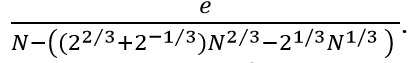# CRYPTANALYSIS OF RSA KEY EQUATION OF N=p^2q FOR SMALL |2q – p| USING CONTINUED FRACTION

## Main Article Content

Muhammad Asyraf Asbullah Normahirah Nek Abd Rahman Muhammad Rezal Kamel Ariffin Siti Hasana Sapar Faridah Yunos

## Abstract

This paper presents a new factoring technique on the modulus , where  and  are large prime numbers. Suppose there exists an integer  satisfies the equation , for some unknown integer  and  is the Euler’s totient function. Our method exploits the term  to be the closest integer to the unknown parameter . Hence we show that the unknown parameters  and  can be recovered from the list of the continued fractions expansion ofFurthermore, we present an algorithm to compute the prime factors of  in polynomial time after obtaining the correct tuple  and.